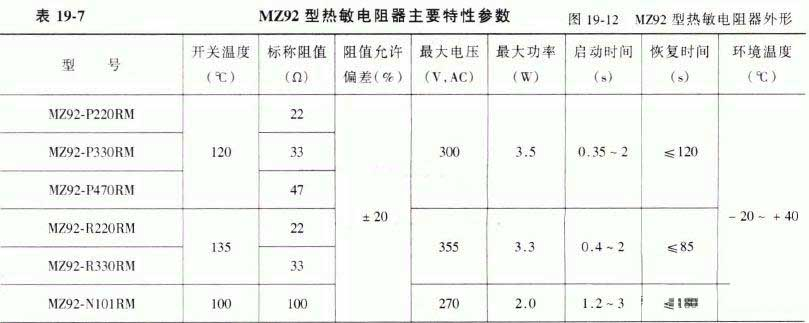﻿ What are the main parameters of the PTC thermistor?_

China sensor manufacturers

# What are the main parameters of the PTC thermistor?

Rated zero power resistor R25
Zero power resistance refers to the very low power consumption added to PTC thermistor when measuring PTC thermistor value at a certain temperature, so that the change of resistance value of PTC thermistor caused by its power consumption can be neglected. The rated zero power resistance refers to the zero power resistance value measured at ambient temperature of 25 C.

Minimum resistance Rmin
Refers to the minimum zero power resistance value that a PTC thermistor can have.

Curie temperature Tc
For PTC thermistor applications, the temperature at which the resistance value begins to increase steeply is important. We define it as the Curie temperature. The resistance of the PTC thermistor corresponding to the Curie temperature RTc = 2*Rmin.

Temperature coefficient α
The temperature coefficient of a PTC thermistor is defined as the relative change in resistance due to temperature changes. The greater the temperature coefficient, the more sensitive the PTC thermistor reacts to temperature changes. α = (lgR2-lgR1)/(T2-T1)

Surface temperature Tsurf
Surface temperature Tsurf: refers to the temperature of the PTC thermistor surface when the PTC thermistor is at a specified voltage and is in thermal equilibrium with the surrounding environment for a long time.

Operating current Ik
The current flowing through the PTC thermistor is sufficient to cause the PTC thermistor to rise above the Curie temperature. The current is called the operating current. The minimum value of the operating current is called the minimum operating current.

Action time Ts
Add an initial current (guaranteed to the operating current) to the PTC thermistor at 25 °C. The time elapsed when the current through the PTC thermistor is reduced to 50% of the starting current is the action time.

No action current INk
The current flowing through the PTC thermistor is insufficient to cause the PTC thermistor to rise above the Curie temperature, which is called a non-operating current. The maximum value of the non-operating current is called the maximum non-operating current.

Maximum current Imax
The maximum current is the highest current withstand capability of the PTC thermistor. The PTC thermistor will fail when the maximum current is exceeded.

Residual current Ir
The residual current is the current in the thermal equilibrium state at the maximum working voltage Vmax.

Maximum operating voltage Vmax
The maximum operating voltage is the maximum voltage that is allowed to remain continuously on the PTC thermistor at the specified ambient temperature. For the same product, the higher the ambient temperature, the lower the maximum operating voltage.

Rated voltage VN
The rated voltage is the supply voltage below the maximum operating voltage Vmax. Usually Vmax = VN + 15%

Breakdown voltage VD﻿
﻿